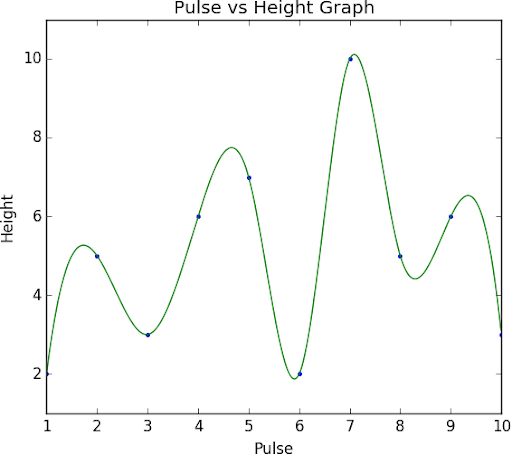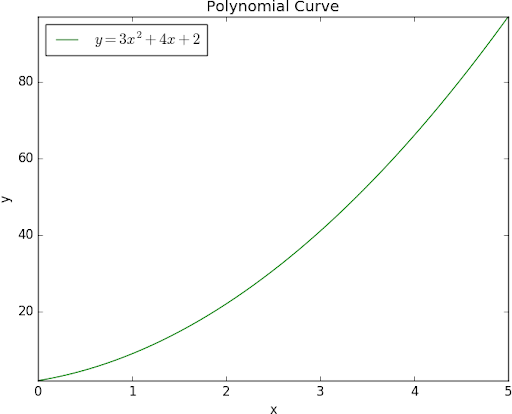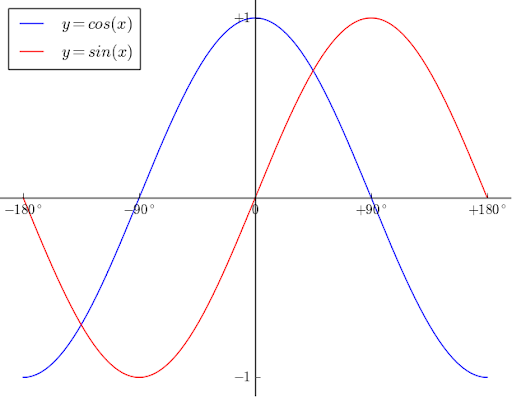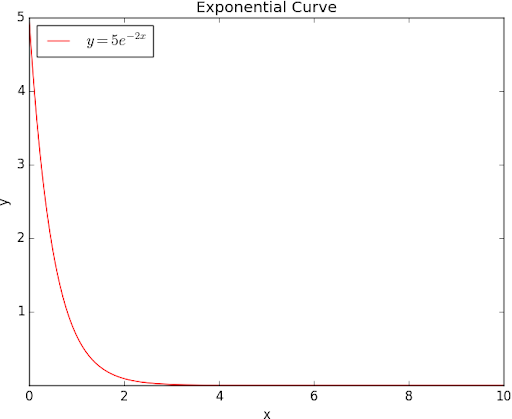## Posts

Showing posts from February, 2014

### Plotting Graph by Keyboard Input using PythonIntroduction to 2D Smooth Graph Connecting line between two points ( x 1 , y 1 ) and ( x 2 , y 2 ) is known as a graph. A non-uniform cartesian co-ordinates points will generate a rigid line, which looks ugly. In Python, a rigid graph can be regenerated into a smooth graph by interpolating more points using SciPy module. SciPy (Scientific Python library) generates more sub-points based on the input array values to form a smooth curve. In this program, I have used input() function to get n number of points ( x , y ) from the user through keyboard ( screenshot is given in the bottom ) and store it to arrays. The co-ordinates points used in the program are (1, 2), (2, 5), (3, 3), (4, 6), (5, 7), (6, 2), (7, 10), (8, 5), (9, 6), and (10, 3). Program Implementation In this tutorial, I have used Python 3.5.2 (64-bit) software, and 7 modules: MatPlotLib 2.0.2 , PyParsing 2.2.0 , Python-DateUtil 2.6.1 , PyTZ 2017.2 , SetupTools 36.2.0 , Cycler 0.10.0 , SciPy 0.19.1 , and NumPy-MKL 1.13

### Polynomial Graph using PythonIntroduction to Polynomial Graph Polynomial curve a is smooth and continues line of graph, connected by a series of co-ordinates calculated using a polynomial equation (For example, y = f ( x ), where f ( x ) = A x 2 + B x + C). In this program, I have used a polynomial equation y = 3 x 2 + 4 x + 2 with x values range from 0 to 5. The program generated co-ordinate points ( x , y ) in the graph will be (0, 2), (1, 9), (2, 22), (3, 41), (4, 66), and (5, 97). Program Implementation In this tutorial, I have used Python 3.5.2 (64-bit) software, and 7 modules: MatPlotLib 2.0.2 , PyParsing 2.2.0 , Python-DateUtil 2.6.1 , PyTZ 2017.2 , SetupTools 36.2.0 , Cycler 0.10.0 , and NumPy-MKL 1.13.1 implemented in Windows 10 Enterprise operating system. The 7 modules are chosen based on the compatibility of Python and OS version and bit. Source Code import numpy as np import matplotlib.pyplot as plt a = 3 b = 4 c = 2 x = np.linspace(0, 10, 256, endpoint = True) y = (a * (x * x)) + (b

### Sine and Cosine Graph using PythonIntroduction to Sine/Cosine Graph The sine and cosine graphs are almost identical, except the cosine curve ( y = cos ( x )) starts at amplitude y = 1, when angle x = 0° (whereas the sine curve ( y = sin ( x )) starts at amplitude y = 0). We say the cosine curve is a sine curve which is shifted to the left by 90°. The graph of sine and cosine is like a wave that forever oscillates between -1 and 1, in a shape that repeats itself every 360° (2π) units. Program Implementation In this tutorial, I have used Python 3.5.2 (64-bit) software, and 7 modules: MatPlotLib 2.0.2 , PyParsing 2.2.0 , Python-DateUtil 2.6.1 , PyTZ 2017.2 , SetupTools 36.2.0 , Cycler 0.10.0 , and NumPy-MKL 1.13.1 implemented in Windows 10 Enterprise operating system. The 7 modules are chosen based on the compatibility of Python and OS version and bit. Source Code import numpy as np import matplotlib.pyplot as plt X = np.linspace(-np.pi, np.pi, 256, endpoint = True) C, S = np.cos(X), np.sin(X) plt.plot(X,

### Exponential Graph using PythonIntroduction to Exponential Graph Exponential curve a is smooth and continues line of graph, connected by a series of co-ordinates calculated using a polynomial equation containing variable exponential value (For example, y = f ( x ), where f ( x ) = A e B x + C). In this program, I have used a polynomial equation with a exponential variable y = 5 e -2 x + 1 with x values range from 0 to 10. The program generated co-ordinate points ( x , y ) in the graph will be (0, 6.0), (1, 1.7), (2, 1.1), (3, 1.0), (4, 1.0), (5, 1.0), (6, 1.0), (7, 1.0), (8, 1.0), (9, 1.0), and (10, 1.0). Program Implementation In this tutorial, I have used Python 3.5.2 (64-bit) software, and 7 modules: MatPlotLib 2.0.2 , PyParsing 2.2.0 , Python-DateUtil 2.6.1 , PyTZ 2017.2 , SetupTools 36.2.0 , Cycler 0.10.0 , and NumPy-MKL 1.13.1 implemented in Windows 10 Enterprise operating system. The 7 modules are chosen based on the compatibility of Python and OS version and bit. Source Code import numpy as np